# Write balanced chemical equations for the following reactions. (a) The reaction of aluminum and iron(III) oxide to form iron and aluminum oxide (known as the thermite reaction). (b) The reaction of carbon and water at high temperature to form a mixture of gaseous CO and H 2 (known as water gas and once used as a fuel). (c) The reaction of liquid silicon tetrachloride and magnesium forming silicon and magnesium chloride. This is one step in the preparation of ultrapure silicon used in the semiconductor industryBuyFind

### Chemistry & Chemical Reactivity

9th Edition
John C. Kotz + 3 others
Publisher: Cengage Learning
ISBN: 9781133949640BuyFind

### Chemistry & Chemical Reactivity

9th Edition
John C. Kotz + 3 others
Publisher: Cengage Learning
ISBN: 9781133949640

#### Solutions

Chapter 3, Problem 1PS
Textbook Problem

## Write balanced chemical equations for the following reactions.(a) The reaction of aluminum and iron(III) oxide to form iron and aluminum oxide (known as the thermite reaction).(b) The reaction of carbon and water at high temperature to form a mixture of gaseous CO and H2 (known as water gas and once used as a fuel).(c) The reaction of liquid silicon tetrachloride and magnesium forming silicon and magnesium chloride. This is one step in the preparation of ultrapure silicon used in the semiconductor industry

Expert Solution

(a)

Interpretation Introduction

Interpretation:

The balanced chemical equation for given reaction should be written.

Concept introduction:

The law of conservation of mass states that no atoms can be created or destroyed in a chemical reaction, therefore, the number of atoms present in the reactants is equal to the number of atoms present in the products.

## Answer to Problem 1PS

Aluminium and iron(III) oxide reacts together and  produces liquid iron and aluminum oxide which is highly exothermic reaction. The balanced equation is given below.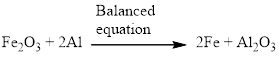### Explanation of Solution

Aluminium and iron(III) oxide reacts together and  produces liquid iron and aluminum oxide which is highly exothermic reaction. The unbalanced equation is given below using correct molecular formula, the given reaction aluminium reduces the oxide of iron.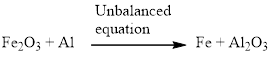Balance the aluminum atom in the given equation, when balancing the equation, we should not alter the subscripts and we can change coefficients.

There is one aluminium in the left side and two aluminium in the right side. Therefore according to the aluminium atom the balanced equation is given below.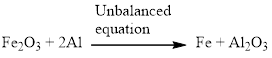Next, balance the iron atom in the equation, there are two iron atom in the left side and one iron atom in right side. For the balancing the iron atom, two molecules of iron is added to the right side. Therefore according to the iron atom the balanced equation is given below.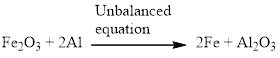Now the equation is balanced, therefore the balanced equation is given below,Expert Solution

(b)

Interpretation Introduction

Interpretation:

The balanced chemical equation for given reaction should be written.

Concept introduction:

The law of conservation of mass states that no atoms can be created or destroyed in a chemical reaction, therefore, the number of atoms present in the reactants is equal to the number of atoms present in the products.

## Answer to Problem 1PS

The balanced equation is given below (b)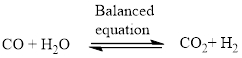### Explanation of Solution

Carbon monoxide reacts with water vapor which forms the carbon dioxide and hydrogen. The mixture of carbon monoxide and hydrogen is called as water gas. This reaction is called as water-gas shift reaction (WGSR).

The unbalanced equation is given below using correct molecular formula, the given reaction Carbon monoxide reacts with water vapor which forms the carbon dioxide and hydrogen, and therefore the balanced equation is given below,Expert Solution

(c)

Interpretation Introduction

Interpretation:

The balanced chemical equation for given reaction should be written.

Concept introduction:

The law of conservation of mass states that no atoms can be created or destroyed in a chemical reaction, therefore, the number of atoms present in the reactants is equal to the number of atoms present in the products.

## Answer to Problem 1PS

Silicon tetrachloride and magnesium reacts together and produces silicon and magnesium chloride. The balanced equation is given below (c)### Explanation of Solution

Silicon tetrachloride and magnesium reacts together and produces silicon and magnesium chloride. The unbalanced equation is given below using correct molecular formula,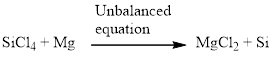Balance the magnesium atom in the given equation, when balancing the equation, we should not alter the subscripts and we can change coefficients.

There is one magnesium atom in the left side and one magnesium in the right side. Therefore according to the magnesium there is no change in the equation. The balanced equation is given below.Next, balance the silicon atom in the equation, there is one silicon atom in the left side and one silicon atom in right side. Therefore according to the silicon atom the balanced equation is given below.Next, balance the chlorine atom in the equation, there is four chlorine atom in the left side and two chlorine atom in the right side. Therefore 2 molecules of magnesium chloride is added to the right side of the reaction. When changing the magnesium chloride the number of magnesium is also changed therefore two molecules of magnesium is added to the left side of the reaction.

Now the equation is balanced, therefore the balanced equation is given below,### Want to see more full solutions like this?

Subscribe now to access step-by-step solutions to millions of textbook problems written by subject matter experts!

### Want to see more full solutions like this?

Subscribe now to access step-by-step solutions to millions of textbook problems written by subject matter experts!© 2021 bartleby. All Rights Reserved.